Courses

# Test: Time And Work- 5

## 30 Questions MCQ Test SSC CGL Tier 2 - Study Material, Online Tests, Previous Year | Test: Time And Work- 5

Description
This mock test of Test: Time And Work- 5 for Quant helps you for every Quant entrance exam. This contains 30 Multiple Choice Questions for Quant Test: Time And Work- 5 (mcq) to study with solutions a complete question bank. The solved questions answers in this Test: Time And Work- 5 quiz give you a good mix of easy questions and tough questions. Quant students definitely take this Test: Time And Work- 5 exercise for a better result in the exam. You can find other Test: Time And Work- 5 extra questions, long questions & short questions for Quant on EduRev as well by searching above.
QUESTION: 1

### A and B can do a piece of work in 30 days, while B and C can do the same work in 24 days and C and A in 20 days. They all work together for 10 days when B and C leave. How many days more will A take to finish the work?

Solution:

2(A + B + C)'s 1 clay's work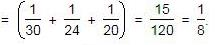Therefore. (A + B + C)'s 1 clay's work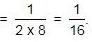Work done by A, B. C in 10 days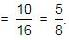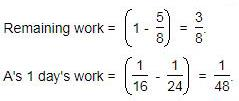Now, 1/48 work is done by A in 1 day.
So. 3/8 work will be done by A in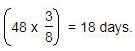QUESTION: 2

Solution:
QUESTION: 3

### Ravi and Kumar are working on an assignment. Ravi takes 6 hours to type 32 pages on a computer, while Kumar takes 5 hours to type 40 pages. How much time will they take, working together on two different computers to type an assignment of 110 pages?

Solution: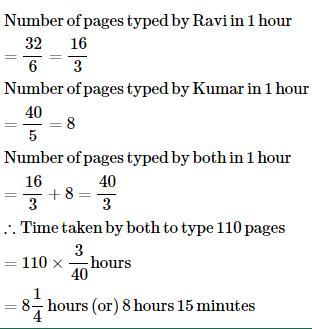QUESTION: 4

A alone can do a piece of work in 6 days and B alone in 8 days. A and B undertook to do it for Rs. 3200. With the help of C, they completed the work in 3 days. How much is to be paid to C?

Solution:
QUESTION: 5

A can do a work in 15 days and B in 20 days. If they work on it together for 4 days, then the fraction of the work that is left is :

Solution:
QUESTION: 6

A and B can complete a work in 15 days and 10 days respectively. They started doing the work together but after 2 days B had to leave and A alone completed the remaining work. The whole work was completed in :

Solution:
QUESTION: 7

X and Y can do a piece of work in 20 days and 12 days respectively. X started the work alone and then after 4 days Y joined him till the completion of the work. How long did the work last?

Solution:
QUESTION: 8

4 men and 6 women can complete a work in 8 days, while 3 men and 7 women can complete it in 10 days. In how many days will 10 women complete it?

Solution:

Let 1 man's 1 day's work = x and 1 woman's 1 day's work = y.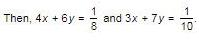Solving the two equations, we get.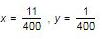∴ 1 woman's 1 day's work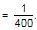⇒ 10 women's 1 day's work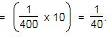Hence. 10 women will complete the work in 40 days

QUESTION: 9

A works twice as fast as B. If B can complete a work in 12 days independently, the number of days in which A and B can together finish the work in

Solution:
QUESTION: 10

A, B and C can complete a piece of work in 24, 6 and 12 days respectively. Working together, they will complete the same work in:

Solution:

A’s 1 day work = 1/24
B’s 1 day work = 1/6
C’s 1 day work = 1/12
(A+B+C)’s 1 day work = 1/24 + 1/6 + 1/12 = 7/24
The work will be completed by them is 24/7 days.

QUESTION: 11

A can finish a work in 18 days and B can do the same work in 15 days. B worked for 10 days and left the job. In how many days, A alone can finish the remaining work?

Solution:
QUESTION: 12

If A and B together can complete a piece of work in 15 days and B alone in 20 days, in how many days can A alone complete the work?

Solution:
QUESTION: 13

A can do a piece of work in 4 hours; B and C together can do it in 3 hours, while A and C together can do it in 2 hours. How long will B alone take to do it?

Solution:

A’s 1 hour work = 1/4
(B + C)’s 1 hour work = 1/3
(C + A)’s 1 hour work = 1/2
(A + B + C)’s 1 hour work = 1/4 + 1/3 =7/12
B’s 1 hour work = 7/12 - 1/2 = 1/12
B alone can do a piece of work in 12 hours.

QUESTION: 14

A machine P can print one lakh books in 8 hours, machine Q can print the same number of books in 10 hours while machine R can print them in 12 hours. All the machines are started at 9 A.M. while machine P is closed at 11 A.M. and the remaining two machines complete work. Approximately at what time will the work (to print one lakh books) be finished ?

Solution:
QUESTION: 15

A can do a certain work in the same time in which B and C together can do it. If A and B together could do it in 10 days and C alone in 50 days, then B alone could do it in:

Solution:

Let a, b and c be the fraction of work per day by A,B & C respectively.
c = 1/50
a + b = 1/10 = 5/50 .
a = b+ c.
Therefore,
2b + c = 5/50
2b = 5/50 - 1/50 = 2/25
b = 1/25
B will do it in 25 days.

QUESTION: 16

A and B together can do a piece of work in 30 days. A having worked for 16 days, B finishes the remaining work alone in 44 days. In how many days shall B finish the whole work alone?

Solution:
QUESTION: 17

If 6 men and 8 boys can do a piece of work in 10 days while 26 men and 48 boys can do the same in 2 days, the time taken by 15 men and 20 boys in doing the same type of work will be:

Solution:
QUESTION: 18

A is thrice as good as workman as B and therefore is able to finish a job in 60 days less than B. Working together, they can do it in:

Solution:
QUESTION: 19

P can complete a work in 12 days working 8 hours a day. Q can complete the same work in 8 days working 10 hours a day. If both P and Q work together, working 8 hours a day, in how many days can they complete the work?

Solution:
QUESTION: 20

A can finish a work in 24 days, B in 9 days and C in 12 days. B and C start the work but are forced to leave after 3 days. The remaining work was done by A in:

Solution:

(B + C)'s 1 day's work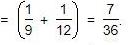Work done by B and C in 3 days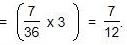Remaining work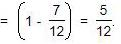Now, 1/24 work is done by A in 1 day.
So, 5/12 work is done by A in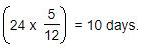QUESTION: 21

A and B can do a work in 8 days, B and C can do the same work in 12 days. A, B and C together can finish it in 6 days. A and C together will do it in :

Solution:
QUESTION: 22

A does 80% of a work in 20 days. He then calls in B and they together finish the remaining work in 3 days. How long B alone would take to do the whole work?

Solution:
QUESTION: 23

A takes twice as much time as B or thrice as much time as C to finish a piece of work. Working together, they can finish the work in 2 days. B can do the work alone in:

Solution:
QUESTION: 24

10 women can complete a work in 7 days and 10 children take 14 days to complete the work. How many days will 5 women and 10 children take to complete the work?

Solution:
QUESTION: 25

A and B can together finish a work 30 days. They worked together for 20 days and then B left. After another 20 days, A finished the remaining work. In how many days A alone can finish the work?

Solution:
QUESTION: 26

Twenty women can do a work in sixteen days. Sixteen men can complete the same work in fifteen days. What is the ratio between the capacity of a man and a woman?

Solution:
QUESTION: 27

A, B and C can do a piece of work in 20, 30 and 60 days respectively. In how many days can A do the work if he is assisted by B and C on every third day?

Solution:
QUESTION: 28

A is 30% more efficient than B. How much time will they, working together, take to complete a job which A alone could have done in 23 days?

Solution:
QUESTION: 29

Sakshi can do a piece of work in 20 days. Tanya is 25% more efficient than Sakshi. The number of days taken by Tanya to do the same piece of work is:

Solution:
QUESTION: 30

X can do a piece of work in 40 days. He works at it for 8 days and then Y finished it in 16 days. How long will they together take to complete the work?

Solution: